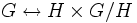Coset representatives and the axiom of choice

This is a survey article related to:left transversal of a subgroup
View other survey articles about left transversal of a subgroup
PREREQUISITES: group, subgroup, left coset of a subgroup, left cosets partition a group, left cosets are in bijection via left multiplication

Consider a group$G$ and a subgroup$H$. Then, the left cosets of$H$ are all of the same size (because left cosets are in bijection via left multiplication) and they partition the group). Thus,$G$ is a union of$|G/H|$ copies of$H$.

This gives Lagrange's theorem:$|G| = |H|[G:H]$.

where$[G:H]$ is the cardinality of$|G/H|$.

However, there is no natural choice of bijection of the form:$G \leftrightarrow H \times G/H$.

Such a bijection can be obtained if we pick a left transversal of$H$ in$G$: in other words, we make a choice of one element from every coset of$H$ in$G$. We first examine how a choice of left transversal yields a bijection.

A left transversal and a coordinatization of the group

How a left transversal gives a bijection with the product

Suppose$T$ is a left transversal of$H$ in$G$. In other words,$T$ contains exactly one element from each left coset of$H$ in$G$. For any$g \in G$, let$t(g)$ denote the representative of the left coset$gH$.

Then, the map is given by:$g \mapsto (t(g)^{-1}g, gH)$.

In other words, the map sends an element of$g$ to the extent by which it differs from its representative, and its coset. The map the other way around is:$(h, gH) \mapsto t(g)h$.

Here is one way of thinking of this. Each coset is a translate of the subgroup, but as a translate, it has no natural origin or reference point with respect to which other elements of the coset can be measured. A choice of representative for each coset gives precisely such a reference point. With this reference point, the left coset can be naturally identified with the subgroup$H$ itself. If we choose a representative for each coset, we thus identify$G$ with the Cartesian product.

Why a left transversal needs the axiom of choice

The axiom of choice is a general result stating that, given an arbitrary family$A_i, i \in I$ of disjoint nonempty sets, we can find a set$B$ such that$B$ intersects each$A_i$ in exactly one element.

To pick a left transversal, we need to do exactly that. We are given an arbitrary collection of disjoint nonempty sets, namely the cosets of a subgroup. We need to pick a left transversal, which is a set that intersects each left coset in exactly one element.

Situations where it does not need the axiom of choice

In many situations, choosing a left transversal does not require the full strength of the axiom of choice:

• When the group is finite, or more generally, when the subgroup has finite index, we only need to make finitely many choices. Making finitely many choices does not require the axiom of choice, and can be done within the framework of axiomatic set theory without choice (Zermelo-Fraenkel set theory).
• In some cases, there is a natural choice of coset representatives. For instance, for the trivial subgroup, there is only one choice of coset representatives. Similarly, for some infinite groups, there is a choice of coset representatives that can be described using a group-theoretic condition.
• When the subgroup has countable index, then choosing a left transversal requires countably many choices. This requires the axiom of countable choice, which is weaker than the full strength of choice. Also, the axiom of countable choice does not lead to any of the paradoxical consequences of the axiom of choice.

Paradoxical consequences of being able to choose coset representatives

The ability to choose coset representatives can have a number of consequences that appear paradoxical. In most of these setups, the group is an uncountable group and the subgroup is countable. The coset representatives are chosen in such a way that they all lie within a compact subset of Euclidean space. This uncountable collection of elements in a compact subset has a very weird arrangement and this arrangement can be used to prove various results.